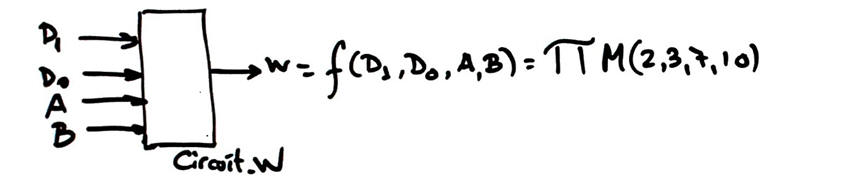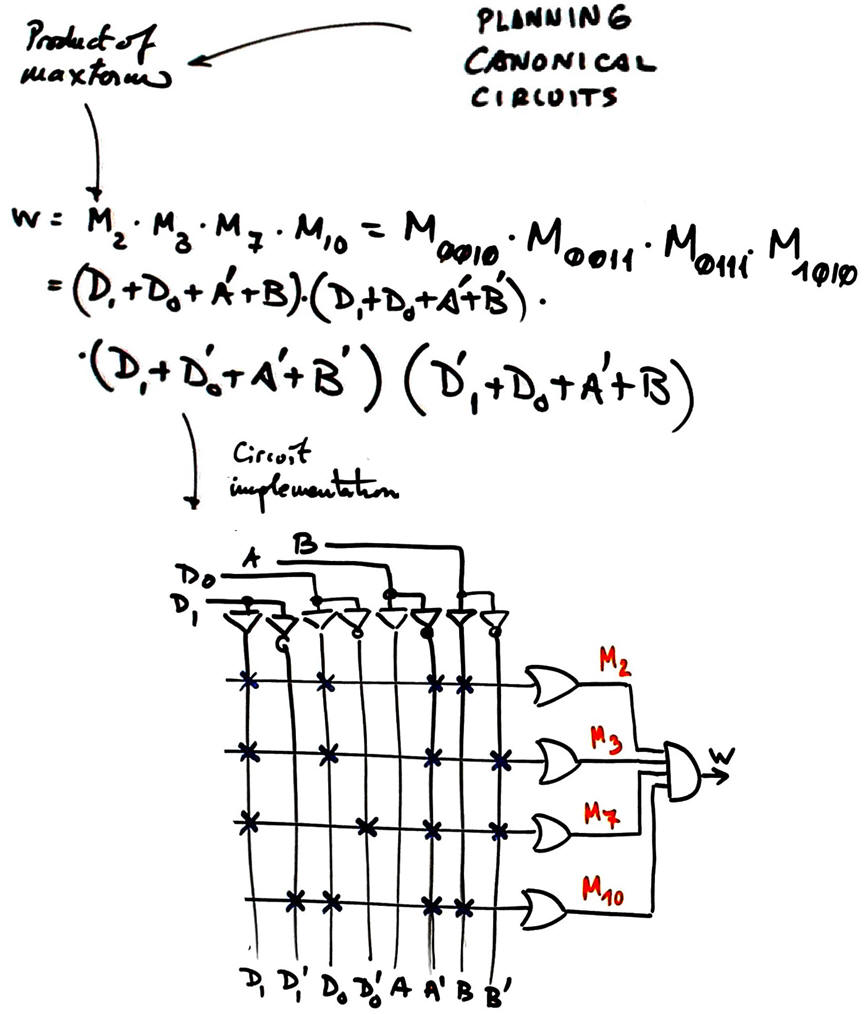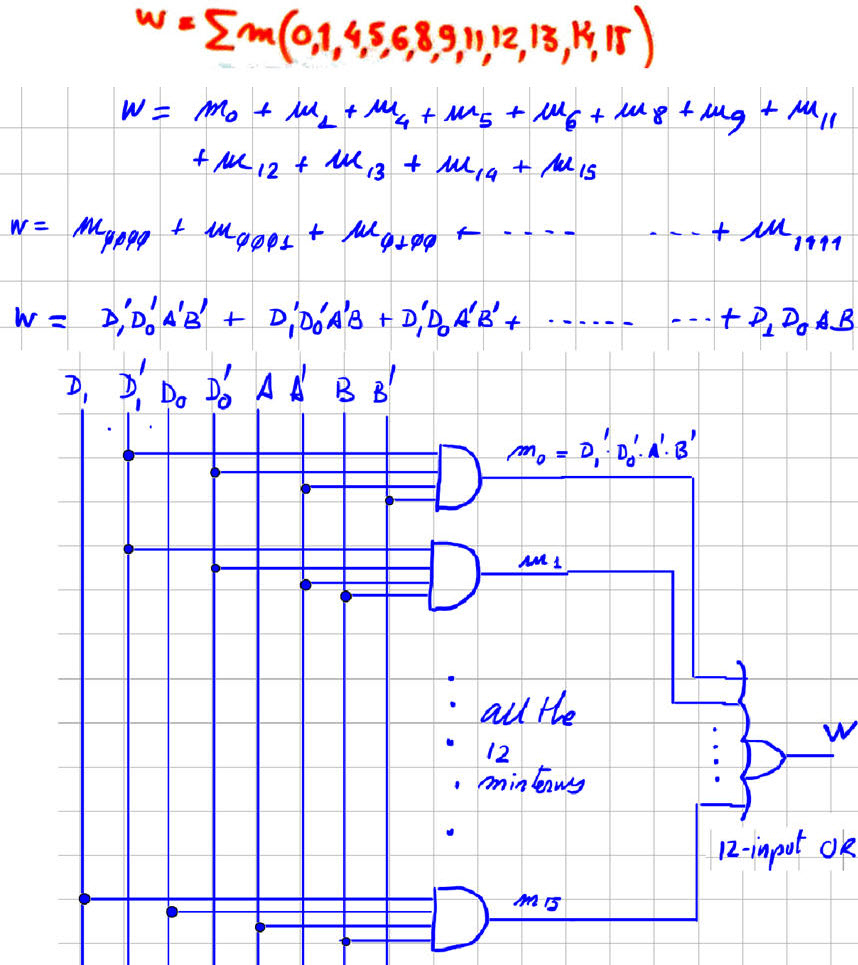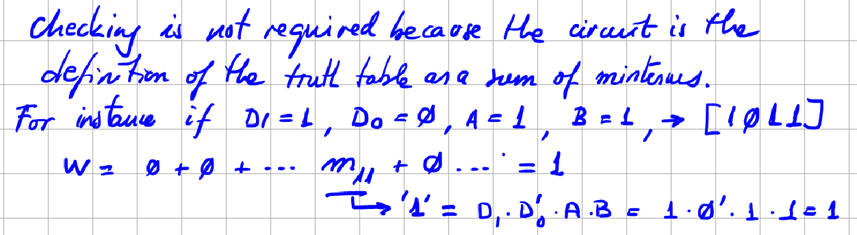﻿ Digital Circuits and Systems - Circuits i Sistemes Digitals (CSD) - EETAC - UPCBachelor's Degree in Telecommunications Systems and in Network EngineeringLecture 4 L1.4: Designing combinational circuits P1 design plan A: canonical circuits, minimisation (minilog), circuits using SoP, PoS [23/2]

1.5. Design flow for inventing combinational circuits using VHDL

1.5.1. Specifications, design concept map (our complete list of design )

1.5.1.1. Symbol or entity

1.5.1.2. Truth table

1.5.1.3. Example timing diagram

1.5.2. CSD design plans

1.5.2.1. Plan A: structural/equations single-file

1.5.2.1.1. Canonical equations: sum of minterms or product of maxterms.

Wikipedia entry for canonical forms.

For example, we can invent four circuits from Circuit_W truth table:Specifications: Invent Circuit_W1 from the truth table of Circuit_W using the canonical expression product of maxterms.Plan and development:

Location for this project files, for instance: pictures, scanned files, report, etc.

C:\CSD\P1\Circuit_W\Circuit_W1\Test: if you test the circuit for example using WolframAlpha or Proteus you will place in this project folder your computer files such as "equations.txt" or "Circuit_W3.pdsprj".

Specifications: Invent Circuit_W2 from the truth table of Circuit_W using the canonical expression sum of minterms.Plan and development:

Location for this project files, for instance: pictures, scanned files, report, etc.

C:\CSD\P1\Circuit_W\Circuit_W2\Test: if you test the circuit for example using WolframAlpha or Proteus you will place in this project folder your computer files such as "equations.txt" or "Circuit_W3.pdsprj". However:1.5.2.1.2. Minimised equations: SoP or PoS

- Espresso heuristic algorithm: minilog.exe (we use Notepad++ as enriched text editor for writing text tables).

- Truth table translated to Minilog text input format (.tbl)

- Minilog minimisation results: SoP or PoS logic equations and equation converter

For example:

Specifications: Invent Circuit_W3 from the truth table of Circuit_W using minimised SoP

Plan: this is the procedure to obtain results and complete the project:

1. Circuit_W3 project location:

C:\CSD\P1\Circuit_W\Circuit_W3\

2. Find and modify a similar tbl file, fr example you can use the file Circuit_P.tbl in minilog tutorial and rename and adapt it to: Circuit_W.tbl

3. Run Minilog and simplify using single output mode (SoM), equation output format, and choose SoP.

4. Equation format that has to be reprocessed by equation_converter.exe so that you get SoP equation with the project entity names for inputs and outputs.

5. Draw the circuit.

6. Check the circuit's equation using WolframAlpha or Proteus.

Development:

- Minilog file describing the truth table: Circuit_W.tblTest: This means verifying that the circuit that you have designed has the given initial truth table. For instance, this is the Proteus capture: Circuit_W3.pdsprj.Specifications: Invent Circuit_W4 from the truth table of Circuit_W using minimised PoS

Plan: (the same as for Circuit_W3)

1. Circuit_W4 project location: C:\CSD\P1\Circuit_W\Circuit_W4\

3. Run Minilog and simplify using single output mode (SoM), equation output format, and choose PoS.

Development.

Test: